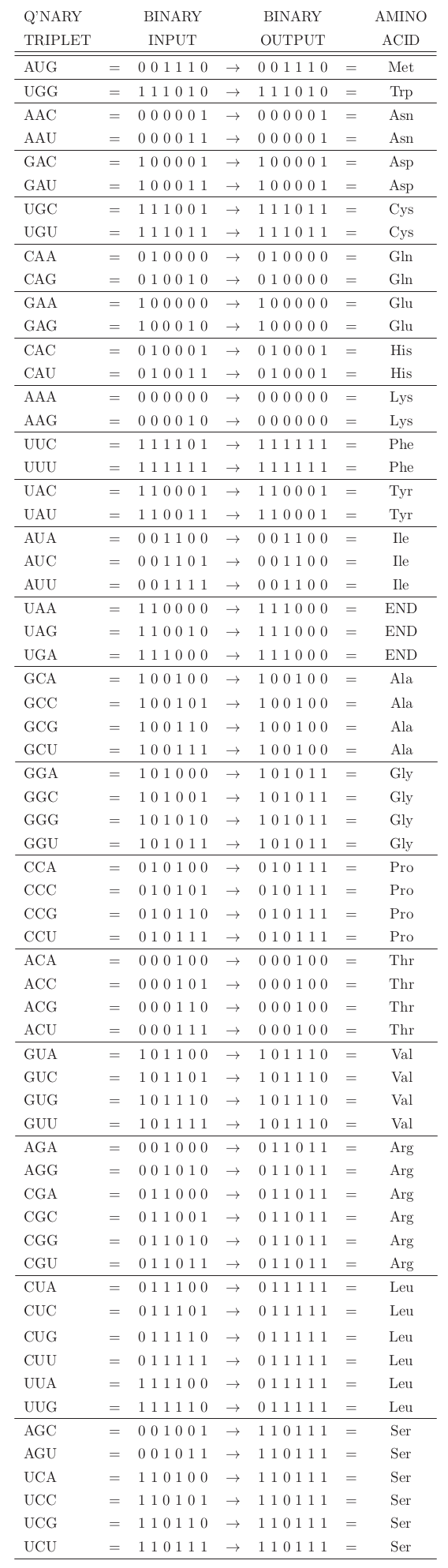An Arkadian Algorithm is any algorithm or group of algorithms which fully describes the protein synthesis process while it adheres to the three theories on the binary nature of the genetic code.

This means the following:

• The Arkadian Algorithm is a hypothetical error correction mechanism that can successfully reduce 64 6-digit binary inputs (from AAA: 000000 to UUU 111111) to just 21 unique outputs.
• 21 inputs are correct (based on the 3rd theory) but 43 inputs are incorrect but the error correction mechanism fixes the errors they contain.
• To build such a mechanism, we need a set of logical rules which we’ll use as a set of components for this mechanism.

As I describe in the book, I used Boolean logic and more specifically the logic of the NAND gate to build an Arkadian Algorithm. The Arkadian Algorithm allowed me to reduce the input into the correct output.

I have started a github project for arkadian algorithms, which shows how we can derive the RNA Codon table programatically. The github address is https://github.com/binaryDNA/arkadian_algorithm

Here is the output table of the Arkadian output described in BINARY NATURE: